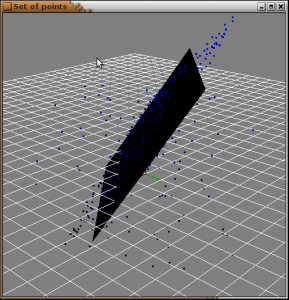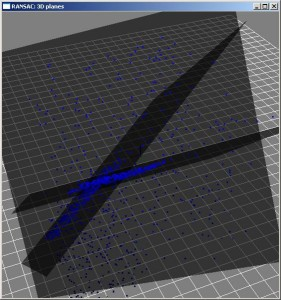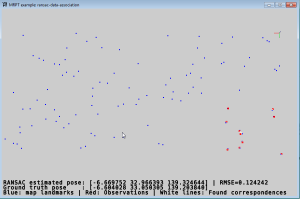# RANSAC C++ examples

## 1. RANSAC algorithm

MRPT comprises a generic C++ implementation of this robust model fit algorithm. For a theoretical description of the algorithm, refer to this Wikipedia article and the cites herein. See also the excellent MATLAB toolkit by Kovesi, on which MRPT’s implementation is strongly based.

The current implementation can be also used to fit a model using both RANSAC and a simple genetic-like modification of it. Refer to the template class mrpt::math::ModelSearch.

## 2. C++ Examples

A few sample applications of RANSAC are provided with MRPT. The generic implementation can be found in the class mrpt::math::RANSAC_template, whose main method is:

For the complete documentation of this class, please see mrpt::math::RANSAC_Template<>.

A complete example of how to use this templatized version can be found in: https://github.com/MRPT/mrpt/tree/master/samples/math_ransac_plane3d_example

There exist as well other application-specific methods (like the N-planes detector), which instantiate the generic template to offer a more user-friendly interface for each specific problem. See mrpt::math::ransac_detect_3D_planes and the “RANSAC detectors” module, part of the library mrpt-base.

### 2.1 Fit a 3D plane

The source for this example can be found in: https://github.com/MRPT/mrpt/tree/master/samples/math_ransac_plane3d_example

This is the resulting fit plane. Average execution time for 300 inliers and 100 outliers is 0.5 milliseconds on a Pentium M @ 2.0Ghz.### 2.2 Fit a number of 3D planes

The source for this example can be found in https://github.com/MRPT/mrpt/tree/master/samples/math_ransac_examples. The method tries to identify an unknown number of planes in a point cloud, given a threshold for considering a point as an inlier and a minimum number of inliers to consider a plane hypothesis as valid. Average execution time for 3 planes x 300 inliers and 300 outliers is 60ms on a Pentium M @ 2.0Ghz.### 2.3 Fit a number of 2D lines

The source for this example can be found in https://github.com/MRPT/mrpt/tree/master/samples/math_ransac_examples.
These are the resulting fit lines.

### 2.4. Data association with RANSAC